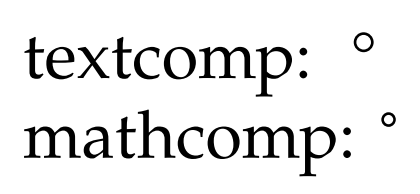# TeX Gyre Pagella degree symbol in math mode?

The textcomp package provides access to the degree symbol in text mode. If I'm understanding it correctly, the mathcomp package purports to provide access to the same in math mode via the \tcdegree command. However, when I use the TeX Gyre Pagella math font, the text-mode \textdegree comes out significantly larger than the math-mode tcdegree. Is there a good way to get the larger degree symbol in math mode? unicode-math will allow me to use \textdegree in math mode, if I ignore the errors that the console throws at me, but that seems like it would be undesirable. Is there a better way?

As an aside, is there a reason the one is larger than the other? Are they distinct glyphs? Is there a typographical reason to use one rather than the other in particular situations?

\documentclass{article}
\usepackage{textcomp}
\usepackage{mathcomp}
\usepackage{tgpagella}

\begin{document}

\noindent
textcomp: \textdegree\\
mathcomp: $\tcdegree$

\end{document}\newcommand\degree{\text{\textdegree}} provides universal (text/math) \degree. Needs \usepackage{amsmath}.

A package like siunitx gives also access to the universal degree symbol, in math mode as well as in text mode.

Note that you have to use XeLaTeX or LuaLaTeX if you want to use the TeX Gyre Pagella math fonts: they are only available in the OpenType format.

\documentclass{article}
\usepackage{siunitx}
\usepackage{unicode-math}
\setmainfont{TeX Gyre Pagella}
\setmathfont{TeX Gyre Pagella Math}
\begin{document}
\SI{30}{\degree}
$\SI{30}{\degree}$
\end{document}